# 1 Starting Out: Elementary Arithmetic

 1 Starting Out: Elementary Arithmetic
As a first example of how the Wolfram Language operates, let’s look at elementary arithmetic.
2 + 2

1234 + 5678

Multiply numbers:
1234*5678

 2+2 addition 5-2 subtraction 2*3 multiplication (2 3 also works) 6/2 division 3^2 raising to a power (e.g. squaring)
1.1Compute 1+2+3. »
Expected output:
 Out[]=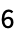Answer & check your solution
1.2Add the numbers 1, 2, 3, 4, 5. »
Expected output:
 Out[]=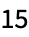Answer & check your solution
1.3Multiply the numbers 1, 2, 3, 4, 5. »
Expected output:
 Out[]=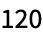Answer & check your solution
1.4Compute 5 squared (i.e. 5×5 or 5 raised to the power 2). »
Expected output:
 Out[]=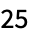Answer & check your solution
1.5Compute 3 raised to the fourth power. »
Expected output:
 Out[]=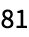Answer & check your solution
1.6Compute 10 raised to the power 12 (a trillion). »
Expected output:
 Out[]=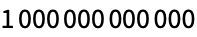Answer & check your solution
1.7Compute 3 raised to the power 7×8. »
Expected output:
 Out[]=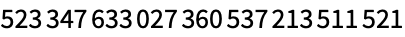Answer & check your solution
1.8Add parentheses to 4-2*3+4 to make 14. »
Expected output:
 Out[]=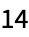Answer & check your solution
Expected output:
 Out[]=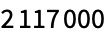Answer & check your solution
+1.1Add all integers from -3 to +3.  »
Expected output:
 Out[]=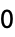Answer & check your solution
+1.2Compute 24 divided by 3. »
Expected output:
 Out[]=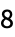Answer & check your solution
+1.3Compute 5 raised to the power 100. »
Expected output:
 Out[]=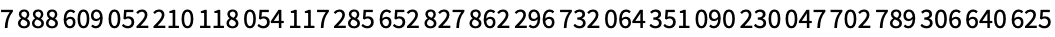Answer & check your solution
+1.4Subtract 5 squared from 100. »
Expected output:
 Out[]=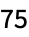Answer & check your solution
+1.5Multiply 6 by 5 squared, and add 7. »
Expected output:
 Out[]=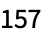Answer & check your solution
+1.6Compute 3 squared minus 2 cubed.  »
Expected output:
 Out[]=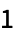Answer & check your solution
+1.7Compute 2 cubed times 3 squared. »
Expected output:
 Out[]=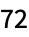Answer & check your solution
+1.8Compute “double the sum of eight and negative eleven”. »
Expected output:
 Out[]=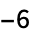Answer & check your solution
In 2+2, etc. how do I tell the Wolfram Language that I’ve finished my input?
Why is multiplication indicated by *?
Because the * (“star”, typically typed as shift+8) looks like a multiplication sign. In the Wolfram Language, you can also just put a space between numbers you want to multiply; the Wolfram Language will then automatically insert a × multiplication sign.
What does “raised to the power” (^) mean?
6^3 means 6×6×6 (i.e. 6 multiplied by itself 3 times); 10^5 means 10×10×10×10×10; etc.
How big can numbers get in the Wolfram Language?
As big as you wantso long as they fit in your computer’s memory.
What is the order of operations in the Wolfram Language?
The same as in ordinary math: powers, multiplication, addition. So 4*5^2+7 means (4*(5^2))+7. You can use parentheses just like in math. (In math, people sometimes use [...] as well as (...). In the Wolfram Language [...] means something different.)
When I do division, how do I avoid getting fractions in my answer?
If you put in numbers with decimal points, you’ll get out numbers with decimal points. You can also use N, as we discuss in Section 23.
What are the little spaces between digits in results like 7006652?
They’re there to help you read the number when it’s displayed; they’re not part of the number.
How do I enter a big number?
Just type the digits, without putting any commas, spaces or other separators (e.g. 1234123511415223).
What happens if I compute 1/0?
Try it! You’ll get a symbolic representation of infinity, on which the Wolfram Language can then do further computations.